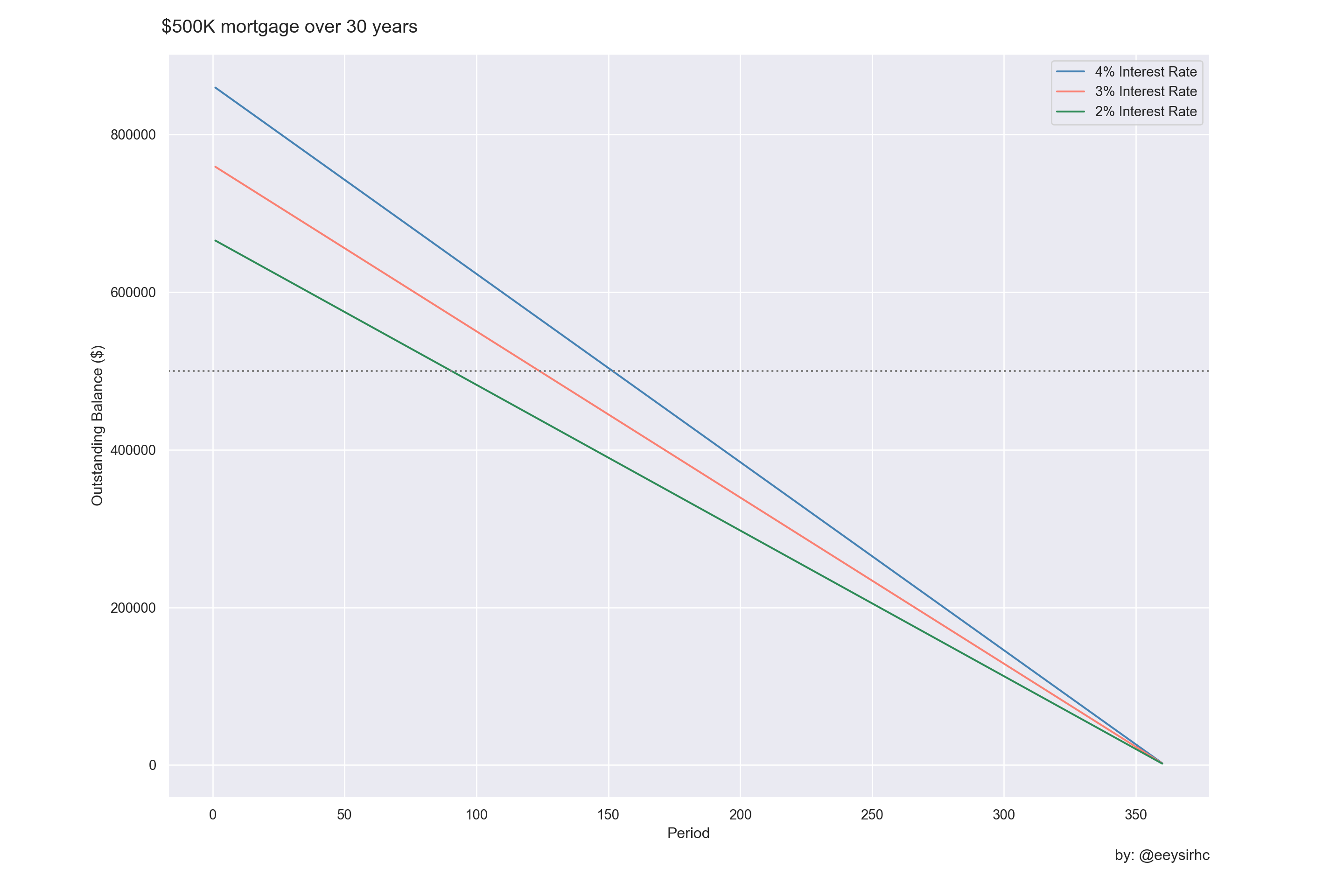With mortgage rates at a historical low there are inklings the US housing market is heating up again. Buying a home is a huge decision and in a perfect world everyone weighs their options and makes a (relatively) rational choice.

One approach is to lay out all the mortgage offers and compare how much more we’re paying over the life of the loan. In this article I want to achieve a few things:

• Create a loan amortization table
• Visualize and compare the different scenarios
• Develop everything in python

``````import pandas as pd
import numpy as np
import matplotlib.pyplot as plt
import seaborn as sns

sns.set(style="darkgrid")``````

## Define function

To build our amortization schedule we can write a python function to accept three arguments: interest rate, mortgage amount, and loan duration.

``````def amortization_schedule(input_intrate, mortgage, years):

##### PARAMETERS #####
# CONVERT MORTGAGE AMOUNT TO NEGATIVE BECAUSE MONEY IS GOING OUT
mortgage_amount = -(mortgage)
interest_rate = (input_intrate / 100) / 12
periods = years*12
# CREATE ARRAY
n_periods = np.arange(years * 12) + 1

##### BUILD AMORTIZATION SCHEDULE #####
# INTEREST PAYMENT
interest_monthly = npf.ipmt(interest_rate, n_periods, periods, mortgage_amount)

# PRINCIPAL PAYMENT
principal_monthly = npf.ppmt(interest_rate, n_periods, periods, mortgage_amount)

# JOIN DATA
df_initialize = list(zip(n_periods, interest_monthly, principal_monthly))
df = pd.DataFrame(df_initialize, columns=['period','interest','principal'])

# MONTHLY MORTGAGE PAYMENT
df['monthly_payment'] = df['interest'] + df['principal']

# CALCULATE CUMULATIVE SUM OF MORTAGE PAYMENTS
df['outstanding_balance'] = df['monthly_payment'].cumsum()

# REVERSE VALUES SINCE WE ARE PAYING DOWN THE BALANCE
df.outstanding_balance = df.outstanding_balance.values[::-1]

return(df)``````

## Build scenarios

Let’s assume a \$500k loan for a standard length of 30 years with a few varying interest rates.

``````input_loan = 5e5
input_years = 30

scenario1 = amortization_schedule(4.00, input_loan, input_years)
scenario2 = amortization_schedule(3.00, input_loan, input_years)
scenario3 = amortization_schedule(2.00, input_loan, input_years)``````

## Visualize

``````plt.figure(figsize=(15,10))

sns.lineplot(x='period', y='outstanding_balance', data=scenario1, color='steelblue');
sns.lineplot(x='period', y='outstanding_balance', data=scenario2, color='salmon');
sns.lineplot(x='period', y='outstanding_balance', data=scenario3, color='seagreen');
plt.axhline(y=5e5, linestyle=':', color='grey')

plt.xlabel("Period")
plt.ylabel("Outstanding Balance (\$)")
plt.suptitle("\$500K mortgage over 30 years", x=0.12, horizontalalignment="left", fontsize=15)
plt.figtext(0.9, 0.04, "by: @eeysirhc", horizontalalignment="right")
plt.legend(labels=['4% Interest Rate', '3% Interest Rate', '2% Interest Rate'])

plt.show()``````The great thing about this plot is how quickly we can identify the contrast between our different loan offers.

Reviewing the first scenario with 4% interest rate reveals we are paying almost +72% over the life of the entire loan, or an extra \$359K in interest.

``scenario1[:1]['outstanding_balance'] - input_loan``
``````## 0    359347.531838
## Name: outstanding_balance, dtype: float64``````

In fact, for this option the original loan amount doesn’t start paying down until ~13 years later:

``scenario1[scenario1['outstanding_balance'] < input_loan][:1]``
``````##      period     interest   principal  monthly_payment  outstanding_balance
## 151     152  1196.352798  1190.72368      2387.076477        498898.983761``````

For comparison the 2% interest rate scenario occurs by year ~8:

``scenario3[scenario3['outstanding_balance'] < input_loan][:1]``
``````##     period    interest    principal  monthly_payment  outstanding_balance
## 90      91  669.256966  1178.840398      1848.097363         498986.28813``````

## Wrapping up

With a little help from the python programming language we were able to build our own amortization schedule, visualize the remaining balance during the life of the loan, and quickly compare & contrast an assortment of mortgage options.

If you found this useful or interesting then please do not hesitate to share with others on your favorite social media platform!